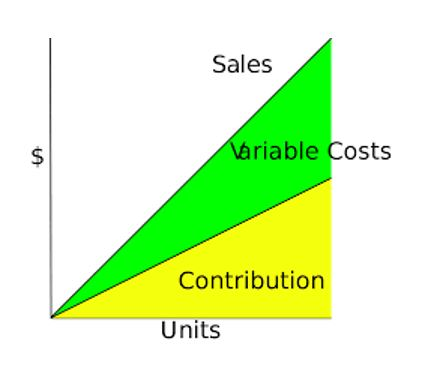# The Contribution Margin: A Tool for Decision MakingMany operations decisions in the production or manufacturing industry are based on how the contribution margin of each product will be affected.

Contribution margin is sales revenue less variable cost. It is the amount of revenue that remains after you have taken out the variable cost of the product. In other words, it is the amount available to cover for the fixed costs and contributes towards profits for the business.

Contribution Margin = Sales revenue – Variable costs

To demystify the concept of contribution margin, let us look at the scenario of a fictional local food vendor, who only sells meals of either Jollof Rice mixed with Beans or boiled Yam mixed with Beans, with a sachet of ‘Pure-water’ and a piece of meat to go with each meal served. Assume each meal is sold at a price of ₦300. Let us also assume the following cost figures for the business:

Fixed cost of setting up the shop for the day (including logistics) = ₦2000

Cost of ‘Pure-water sachet’ = ₦10

Cost of piece of Meat = ₦15

Cost of boiled Beans = ₦60

Cost of Jollof-Rice = ₦80

Cost of Boiled Yam = ₦100

Cost of Sauce = ₦30

Variable cost for Jollof Rice and Beans meal will be = 80 + 60 + 15 + 10 + 30 = ₦195

Variable cost for boiled Yam and Beans meal will be = 100 + 60 + 15 + 10 + 30 = ₦215

Since the meals are sold at the same price of N300 per meal, the Contribution margin (CM) for each meal type is calculated as follows:

Contribution margin for Jollof Rice & Beans meal = ₦300 – ₦195 = ₦105

Contribution margin for Boiled Yam and Beans = ₦300 – ₦215 = ₦85

Assume that, based on received orders, the food vendor was to sell only Jollof Rice & beans meal for the day, he must sell 19 meals to cover his daily fixed cost of ₦2000. That is, 2000 ÷105 = 19.

If on the other hand, meal request for the day were only boiled Yam and Beans, he must sell 24 meals to cover the daily fixed cost. That is, 2000 ÷ 85 = 24.

Taking this further, since Jollof Rice & Beans meal offers a higher contribution margin of ₦105, the food vendor should endeavor to sell more of the Jollof Rice & Beans meal.

Understanding the concept illustrated in the above scenario, helps to understand the breakeven concept.

Going back to the local food vendor scenario; the number of meals calculated to cover the daily fixed cost is also called the breakeven point.

That is, (fixed cost) ÷ (contribution margin) = breakeven point.

If the food vendor decides to make a profit of ₦1500 at the close of business for the day, all he needs to do is add ₦1500 to the fixed cost before carrying out the same calculations we did earlier:

For instance, with the case of selling only Jollof Rice and Beans, the number of meals to cover both the fixed cost and a profit of ₦1500 will be:

₦3500 ÷ ₦105 = 33 meals

(Fixed cost + desired profit) ÷ Contribution margin = number of units/products to be sold to yield revenue that will cover fixed cost and meet the profit target.

In conclusion, the above explanations also provide clarity on the relationship between Sales revenue/price, the fixed cost, the variable cost, and the desired profit.

Profit = Sales revenue – fixed cost – variable cost.

Depending on your target profit value, with the above equation, you can analyze various cost related decision alternatives before choosing one.

Thanks for reading and hope you now have a better grasp of the contribution margin concept.

#MEMBA11## UNDERSTANDING THE RELATEDNESS OF FINANCIAL STATEMENTS OF AN ORGANISATION: A USEFUL TOOL IN PERFORMANCE EVALUATION.

in## Ghostwriting

in